Courses
Courses for Kids
Free study material
Free LIVE classes
More

# JEE Advanced 2023 Solid State Revision NotesLIVE
Join Vedantu’s FREE Mastercalss

Solids are different from fluids in various aspects. The molecules of a solid remain in a highly compacted state. The intermolecular attraction in the solids is the highest causing no free flow of the molecules. This is why they have a specific volume and shape. Solid State is an important chapter covered in the JEE Advanced syllabus. To understand the various concepts of this chapter, go through the JEE Advanced Solid State revision notes prepared by the subject experts at Vedantu.

These Solid State JEE notes have been prepared as per the latest JEE syllabus to offer a better understanding of the advanced concepts. Students will be able to learn the theories and principles of Solid State here and resolve their doubts on their own.

Last updated date: 20th Sep 2023
Total views: 138.3k
Views today: 1.38k

## JEE Advanced Revision Notes Chemistry Solid State

In crystalline solid atoms, ions or molecules are arranged orderly in a three-dimensional manner due to strong binding force.

Due to this arrangement, crystalline solids acquire rigidity and definite geometric shape or crystalline structures.

### Characteristics Properties of the Solids

• They have definite mass, volume and shape.

• Intermolecular distance is short.

• Intermolecular forces are strong.

• Their constituent particles (atom, molecules or ions) have fixed positions and can only oscillate about their mean position.

• Their existence of a substance in more than one solid modification is called Polymorphism.

Anisotropy: Crystalline solids are anisotropic in nature that is some of their physical properties like electrical resistance of refractive index show different values when measured along with different directions in the same crystals. This arises from the different arrangements of particles in different directions.

Isotropy: Amorphous solids on the other hand are isotropic in nature, it is because there is no long-range order in them and arrangement is irregular along with all the directions. Therefore, the value of any physical property would same in any direction.

Annealing: An amorphous substance, on heating at a certain temperature, may attain crystalline nature while on cooling regains its amorphous nature, this phenomenon is called annealing.

### Difference Between Crystalline and Amorphous Solids:

 Property Crystalline solids Amorphous solids Shape Definite characteristics geometrical shape. Irregular shape. Melting point Melt at a sharp and characteristic temperature. Gradually soften over a range of temperature. Cleavage property When cut with a sharp-edged tool, they split into two pieces that are plain and smooth. When cut with a Sharpe-edged tool, they cut into two pieces with an irregular surface. Heat of fusion They have definite and characteristics value. They do not have definite value. Anisotropy Anisotropic in nature except for cubic crystals. Isotropic in nature. Nature True solids Pseudo solids or super cooled liquids Order in arrangement of constituent particles Long range order Only short-range order Law of crystallography Follows Does not follow X-ray diffraction bands Forms Does not form Cooling Curve Cooling curve is not smooth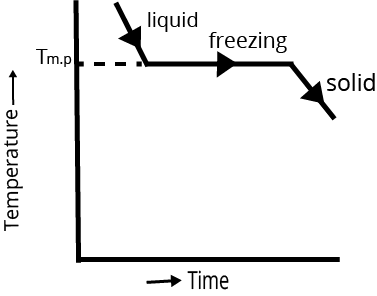Cooling curve is smooth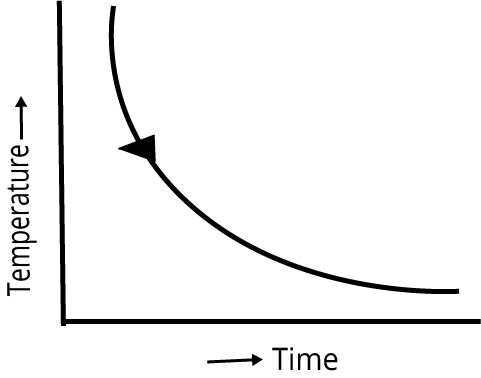Example NaCl, Diamond, MgO Glass, Rubber, Plastic, Quartz glass

### Classification of Crystalline Solids:

A crystal is classified as ionic, covalent, metallic and molecular according to the nature of the building units, chemical bonding and the intermolecular forces in the crystal.

### Different Types of Solids:

 S.No Types of Solid Constituting Unit Interparticle Force Example Physical Mode Electrical Properties. 1 Molecular solids i) polar-molecular solid. Polar molecules Dipole, dipole attraction forces HCl, $S{O_2}$etc Soft solid with low melting point Insulator ii) Non-polar molecular solids Non -polar molecules van der Waals’s force of attraction $BeC{l_{2,}},CC{l_4}$ Soft solid with  very low melting point Insulator iii) H-bonding solids Molecules capable to from H-bond H-bonds $N{H_4}$,ice, glucose, etc Harder than (i) and (ii) with low melting point Insulator 2 Covalent or network solids. Atoms Covalent bonds Diamond, quartz, silica Very hard melting point rigid Insulator/Conductor 3 Ionic solids Cations and anions Electrostatic force of attraction NaCl, Zns Hard rigid high m.pt Conductor in fused state 4 Metallic solids Metal atom(kernels embedded in sea of electrons) Metallic bonds Metal Cu, Ag, Mg, Fe Hard but malleable and ductile conductor

### Law of Crystallography:

The study of structure, geometry, and properties of crystals is called Crystallography.

### Different Law of Crystallography is

1. Law of Constancy of Interfacial Angle:  measured at the same temperature similar angle on crystals of the same substance remains constant regardless of the size and shape of the crystal.

2. Law of Constancy of Symmetry:  The symmetry in all crystals of a particular species is constant though, i.e., the crystals of the same substance possess the same symmetry of element.

### The Symmetry of Elements is:

• Plane of symmetry

• Axis of symmetry

• Centre of symmetry

1. Law of Rational Indices: The law of rational indices states that the intercept of any face of crystal along the crystallographic axes is either equal to the unit intercept or some simple whole number multiples of them.

### Unit Cell

The smallest portion of the crystal lattice which can be used as a repetitive unit in a three-dimension manner to get the entire crystal lattice is called a unit cell.

They are broadly divided into two categories, primitive and centred unit cells.

a. Primitive Unit Cell: when constituent particles are present only in the corner position of a unit cell, it is called a primitive unit cell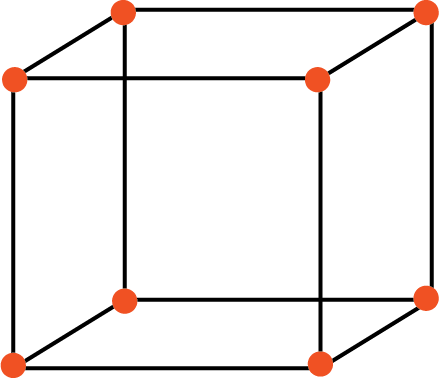Primitive Unit Cell

b. Centred Unit Cell: When a unit cell contains one or more constituent particles present at a position other than corners in addition to those at corners, it is called centred unit cell.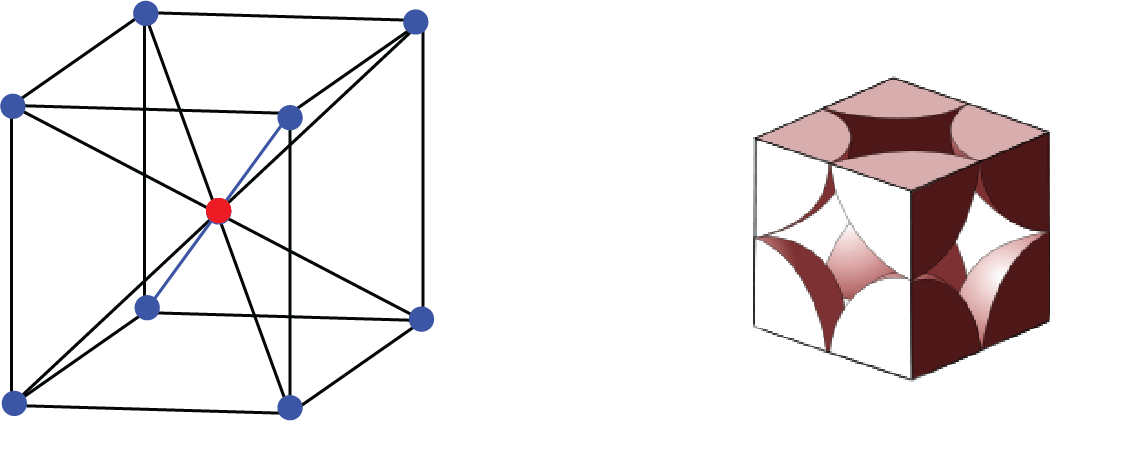Centred Unit Cell

### There are 3 Types:

• Simple cubic cell

• Body-centred unit cell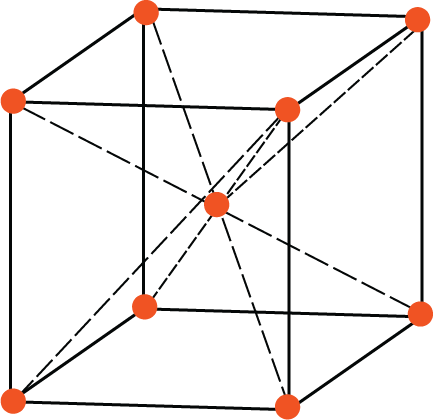Body-Centred Unit Cell

• Face-centred cell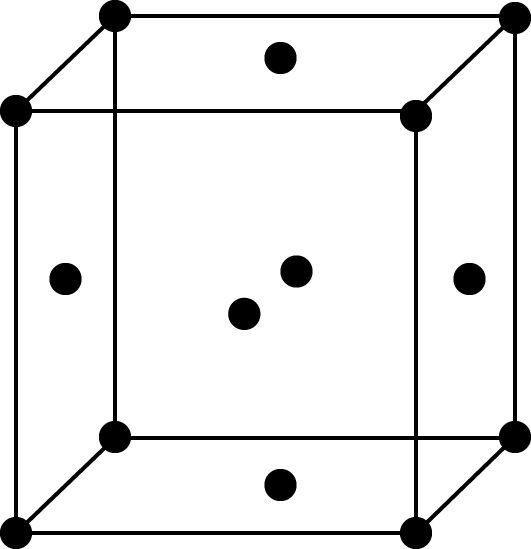Face-Centred Cell

### Bravais Lattices:

• Bravais Lattices showed there are 14 different possible kinds of three-dimensional lattices.

• These 14 Bravais lattices are grouped into seven crystal systems based on unit cell symmetry.

### These Seven Crystal Systems are:

 Crystal System No. of Bravais Lattices Possible Names of These Lattices Cubic 3 Simple cubic, fcc and bcc Tetragonal 2 Primitive body-centred face centre Orthorhombic 4 Primitive body-centred face centre and end centred Hexagonal 1 Primitive Hexagonal prism Trigonal 1 Rhombohedral (or) primitive Monoclinic 2 Primitive and end centred Triclinic 1 Triclinic primitive

### Seven Crystal Systems: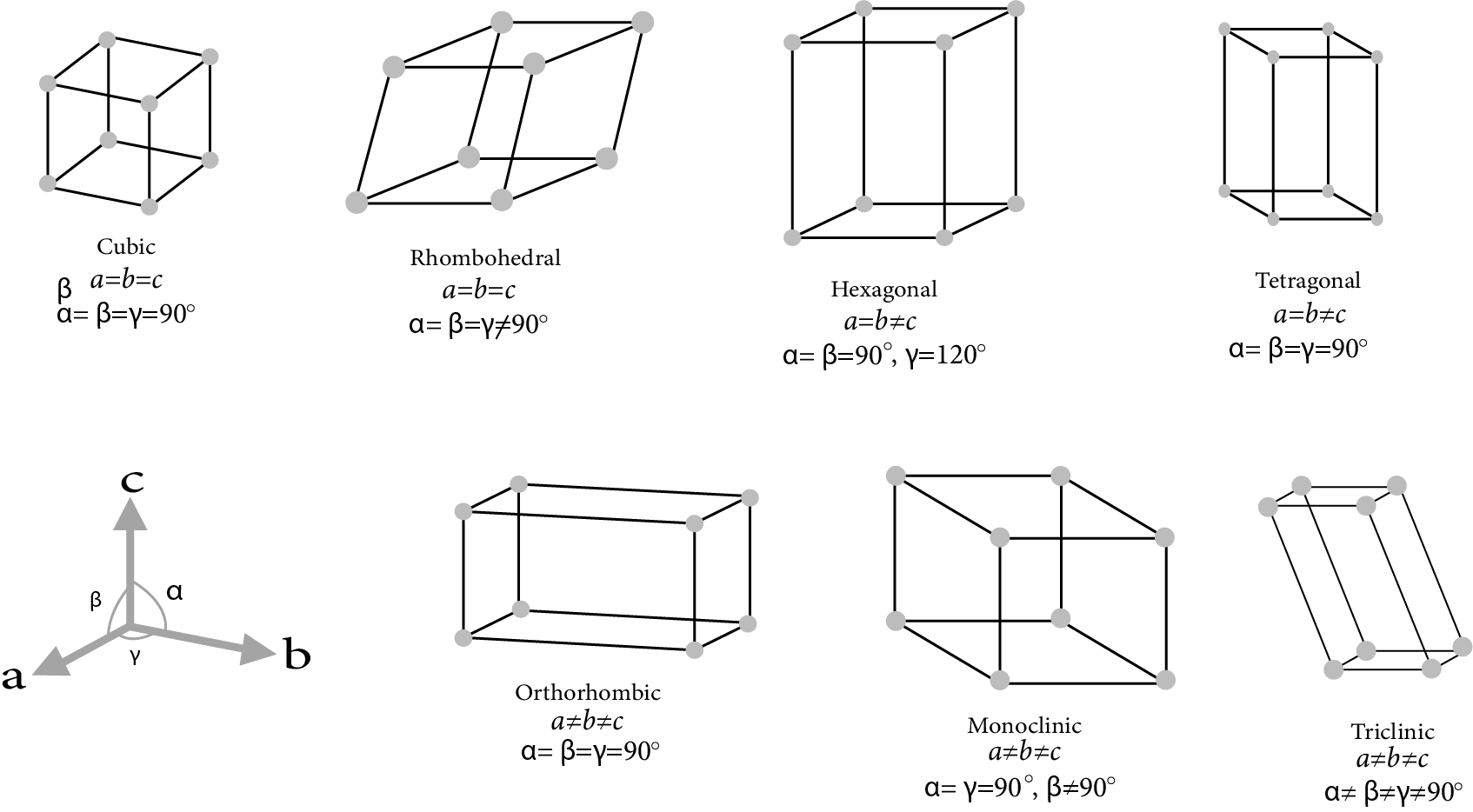Seven Crystal System

• The two tetragonal: one side different length to the other, two angles between face all 90°

• The four orthorhombic lattices: unequal sides, angles between faces all 90°

• The two monoclinic lattices: unequal sides, two faces have angle different to 90°

### Coordination Number for Elemental Crystallization:

The coordination number is the number of nearest such lattice point surrounding a lattice point in a crystalline.

Simple Cubic Cell: In a lattice of this type, each corner atom is shared by eight-unit cells, four in one layer and four in the layer above it.

Thus, the contribution of each atom placed at the corner of the single cubic unit cell is $\dfrac{1}{8} \times 8 = 1$. Thus, the rank of a primitive unit cell is 1.

BCC Unit Cell: In a BCC lattice, each corner atom is shared by 8-unit cells while the body-centred atom is not shared by any other unit cell. So, the number of effective atoms associated with a BCC unit cell is  $\left[ {\dfrac{1}{8} \times 8} \right] + 1 = 2$. Thus, the rank of the BCC unit cell is 2.

FCC Unit Cell: In an FCC unit cell, each corner atom is shared by 8-unit cells and each face centred atom is shared by 2-unit cells, so the number of effective atoms in an FCC unit cell would be $\left[ {\dfrac{1}{8} \times 8} \right] + \left[ {\dfrac{1}{2} \times 6} \right] = 4$, thus the rank of FCC unit cell is 4.

HCP Unit Cell: Each corner atom would be common to 6 other unit cells, therefore their contribution to one unit cell would be $\dfrac{1}{6}$, Total number of atom in 1 hcp cell $= \dfrac{{12}}{6}$(from 12 corners)+$\dfrac{2}{2}$(from 2 face centred)+$\dfrac{3}{1}$(from body centre)=6.

### Hexagonal Closest Packing of Spheres:

• Normal

• Diagrammatic view

Interstitial Voids: The empty spaces between the three-dimensional layers are known as holes or voids. The holes are also referred to as interstices. There are three types of holes possible.

Tetrahedral Holes:  A hole formed by three spheres in contact with each other of a layer. The holes are also referred to as interstices.

Radius ratio of the tetrahedral void,$\dfrac{{{r_{void}}}}{{{r_{sphere}}}} = 0.225$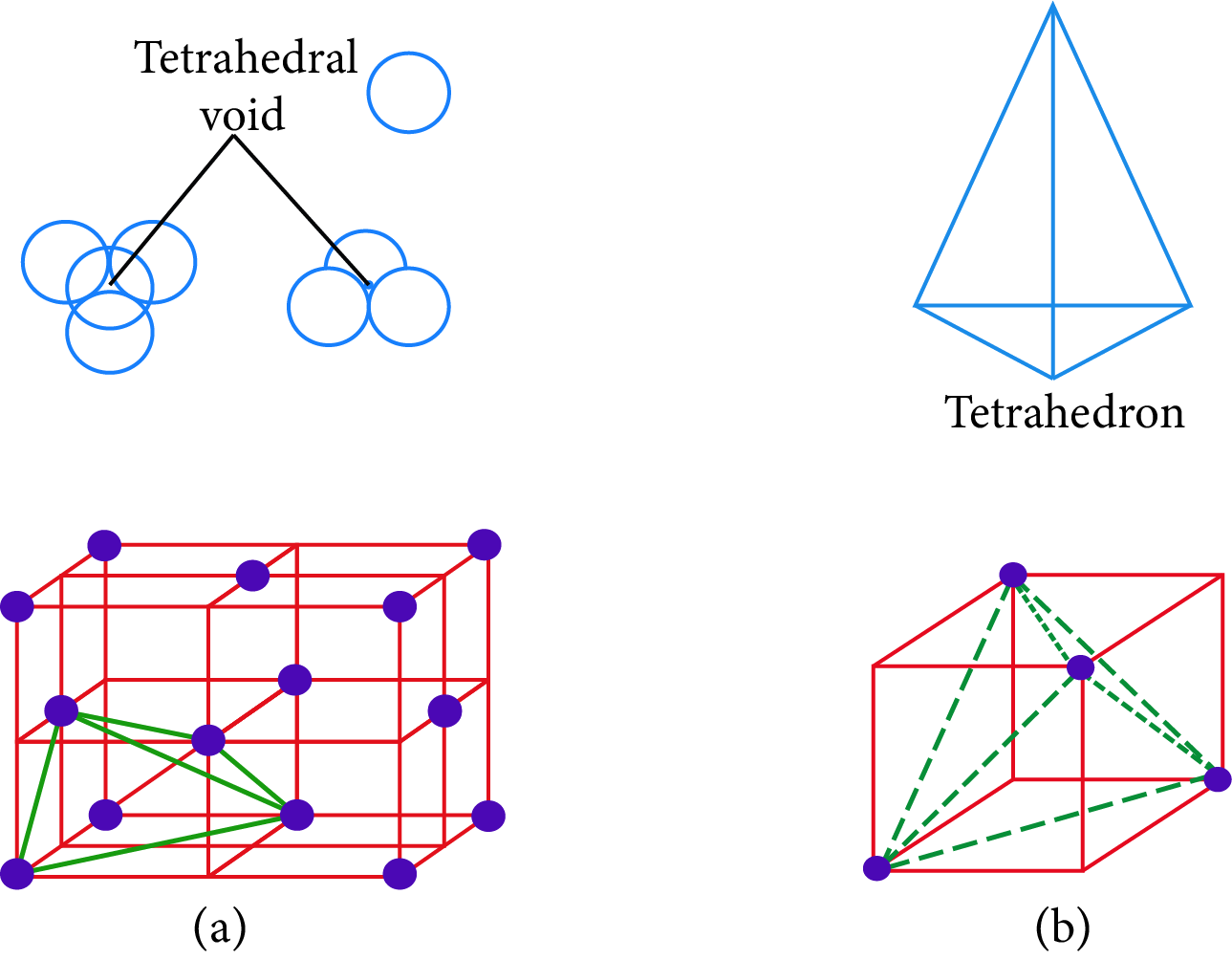Tetrahedral Hole

Octahedral Hole: It is the vacant space between a group of three-sphere in a layer and another set of three-sphere in the next layer, these six spheres surrounding the hole, lie at the vertices of a regular octahedral.

The total number of octahedral voids $= 1 + 12 \times \dfrac{1}{4} = 1 + 3 = 4$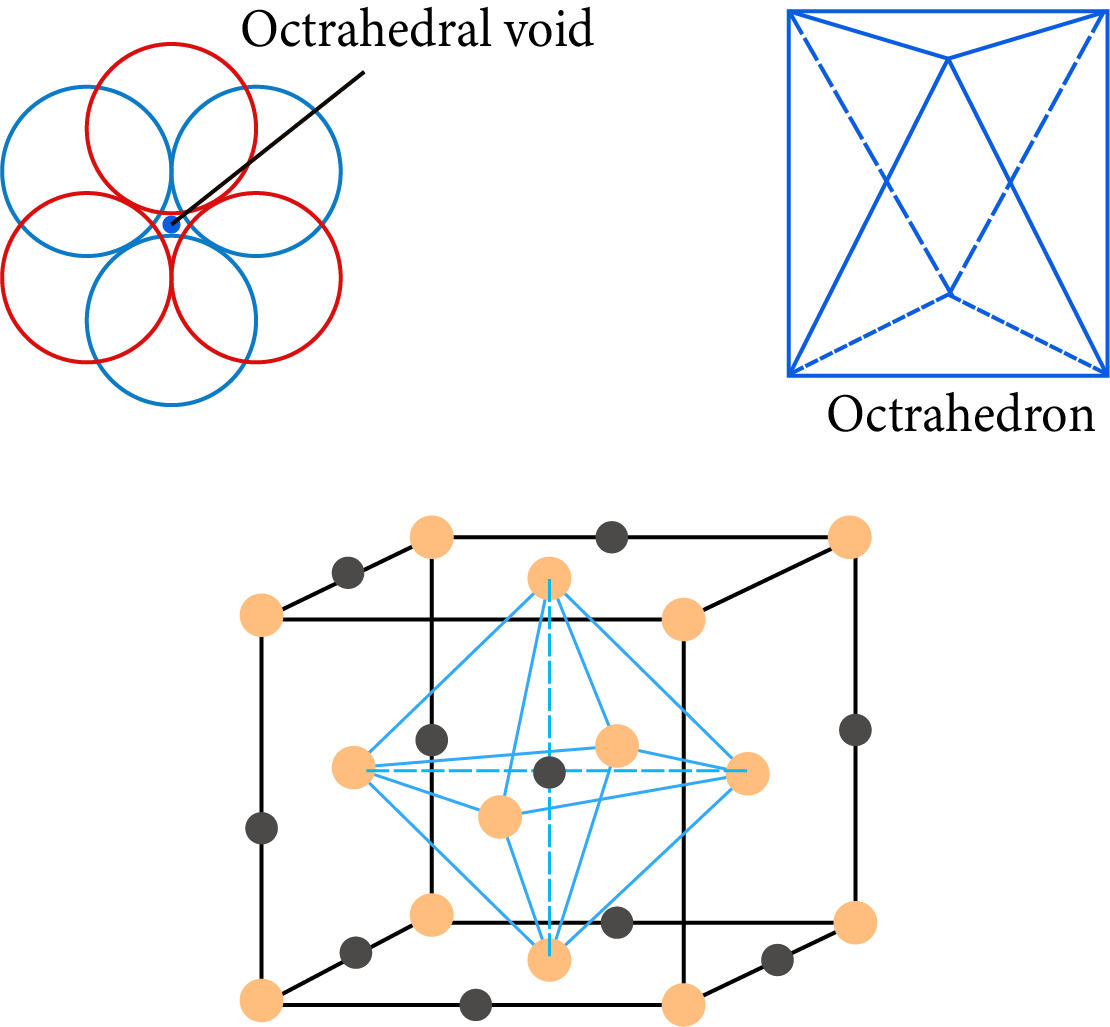Octahedral Hole

### Packing Fraction in Element Crystallization:

• Percentage efficient of simple cubic unit cell =$\dfrac{{{\text{volume of one sphere}}}}{{{\text{volume of unit cell}}}}{\text{ }\times 100}$

${a^3}$= volume of the unit cell

$a = 2{r_s}$ where ${r_s}$ is the radius of the sphere.

$\dfrac{{\dfrac{4}{3}\pi \times {{({r_s})}^3}}}{{{a^3}}} \times 100 = \dfrac{{\dfrac{4}{3}\pi \times {{({r_s})}^3}}}{{{{\left( {2 \times {r_3}} \right)}^3}}} \times 100$

$= \dfrac{{\dfrac{4}{3}\pi }}{8} \times 100 = 52.3$

• Percentage efficiency of body-centred unit cell =$\dfrac{{{\text{volume of the two sphere}}}}{{{\text{Volume of cube}}}}{\text{ }\times 100}$

${a^3}$= volume of the unit cell by taking edge length ‘a’

$a = 2{r_s}$where ${r_s}$ is the radius of the sphere.

$\dfrac{{2 \times \dfrac{4}{3}\pi {{({r_s})}^3}}}{{{a^3}}} \times 100 = \dfrac{{2 \times \dfrac{4}{3}\pi {{({r_s})}^3}}}{{{{\left( {\dfrac{4}{{\sqrt 3 }}{r_s}} \right)}^3}}} \times 100 = 68.05$

The volume occupied by spheres in body-centred unit cell is 68.05% and void volume is 31.95%

Length of facial diagonal -$\sqrt{2a}$

Length of body diagonal -$\sqrt{3}a$

• Efficiency of packing in face-centred unit cell = $\dfrac{{{\text{volume of the four sphere}}}}{{{\text{Volume of cube}}}}{\text{ }\times 100}$

${a^3}$= volume of the unit cell by taking edge length ‘a’

$a = 2{r_s}$where ${r_s}$ is the radius of the sphere.

$\dfrac{{4 \times \dfrac{4}{3}\pi {{({r_s})}^3}}}{{{a^3}}} \times 100 = \dfrac{{4 \times \dfrac{4}{3}\pi {{({r_s})}^3}}}{{{{\left( {2\sqrt 2 {r_s}} \right)}^3}}} \times 100 = 74.06$

Thus, the volume occupied by a sphere in face-centred unit cell (cpp arrangement) is 74.06% and the void volume is 25.94%

• Volume Fraction in HCP:

$V.F = \dfrac{{6 \times \dfrac{4}{3}\pi {R^3}}}{{{V_{HCP}}}}$

${{\text{V}}_{{\text{HCP}}}}{\text{ = Area of base }\times Height}$

${\text{ = 3}}\sqrt {2{a^3}} = 24\sqrt 2 {R^3}$

Volume fraction of HCP=$4\sqrt {\dfrac{2}{3}} R$

Note: Distance between A and B layer in CCP or HCP=$2\sqrt {\dfrac{2}{3}} R$

### Bragg’s Equation: $n\lambda = 2d\sin \theta$

n= order of reflection

$\lambda$=wave length of incident x-ray

d= interplanar distance

$\theta$=angle of reflection

### Types of Crystal Defects:

• Intrinsic Defect: These are seen in pure crystal.

• Extrinsic Defects: These are due to the impurities in the solids.

• Point Defect: These Occur at the lattice points or sites in the crystals.

• Extended Crystal: These are present in one or more dimensions.

### Stoichiometric Defects:

Ionic Solids Show Two Types of Defects

1. Schottky Defect

• This defect arises due to vacancy at cation sites and equal number of vacancies at anion sites

• Condition for the defect:

High co-ordination numbers and where the positive and negative ions are of similar size i.e., $\dfrac{{{r^ + }}}{{{r^ - }}} \cong 1$

• Number of Schottky defect

• $n = N{e^{ - E/2kt}}$

• $n = {\left( {N{N_i}} \right)^{1/2}}{e^{ - E/2kt}}$

K = Boltzmann’s constant

T= Kelvin temperature

E= energy required to create defect

N= number of ions present

Ni= number of interstitial spaces

1. Frenkel Defect

• This type of defect arises due to a vacancy at a cation site.

• Conditions  that favour the defect:

Large difference in size between cation and anion and Compounds having ions of different size i.e., $\dfrac{{{r^ + }}}{{{r^ - }}}$ is low since positive ions are smaller than negative ions.

Doping:

• Addition of B(IIIA Group) or P or As (VA Group) element to alter the conductivity of Ge(or) Si is called Doping.

• Pure Si (or) Ge are intrinsic semiconductor.

• In doping, group IIIA(13 group element) elements behave as electrons acceptor and group VA(15 group elements) elements behave as donor.

## Importance of Physical Chemistry Solid State

Here you will learn the different aspects of the Solid State at an advanced level. This chapter will explain how the molecules form solid and what their characteristics are.

Go through these notes to quickly revise the physical properties of a solid and realize how this state of matter is defined. With the knowledge of the physical properties of different solids, differentiate, distinguish, and identify different materials. This chapter will also describe the classification of solids following the various features.

The importance of this chapter is that it explains the different physical properties of all classes of solids with examples. All these classifications are done based on particular parameters such as crystal structure and chemical bonding. It will also explain how lattices are formed and what kinds of lattices exist with pictorial illustrations. Get Solid State for JEE Advanced key concepts from the chapter and refer to these notes for a quick revision.

## Benefits of Vedantu’s Solid State JEE Notes PDF

• The revision notes offer a simpler explanation of all the advanced concepts of this chapter in an easy-to-understand language. You will find it easier to learn different types of solids, types of crystalline structures, their examples, and all other topics included in this chapter.

• The simpler version of this chapter in the Solid State JEE notes PDF will help you complete preparing this chapter in no time. You will find learning the fundamental principles much easier. In fact, you can also resolve doubts on your own and proceed to solve exercise questions without any difficulty.

• These revision notes are the easiest way to revise the chapter before the exam date. These notes also come with Solid State JEE Advanced questions and answers prepared by the experts. Students can try to solve the questions on their own and check their preparation level.

• Students can easily recall all the concepts, definitions, derivations, formulas, etc of this chapter by using these notes to score well in the competitive exam.

Students can add the free PDF version of these revision notes and questions to their JEE Advanced preparation material for this chapter. Many students prefer to use these notes to make their study sessions more productive. The simpler format of these notes will help them to recall the definitions, explanations, formulas, and derivations of this chapter without any difficulty.

## FAQs on JEE Advanced 2023 Solid State Revision Notes

1. What is perfection in solids?

The crystal structure of a solid remains in perfect order at 0 K. It is called perfection in the solid state. The electrons start to move randomly as the temperature gradually increases. It results in an imperfect stage.

2.  What are insulators?

As per the definition in this chapter, a solid that does not allow electrons or heat energy to pass through is called an insulator. Example: plastic, Bakelite, etc.

3.  What are diamagnetic substances?

These are magnetic substances that are weakly attracted by magnets. They contain paired electrons in the outermost shells. Example: titanium dioxide and sodium chloride.

4.  What is an amorphous solid?

The solids that do not have an even crystalline shape with no sharp edges are called amorphous solids. These solids do not have a proper melting point and heat of fusion.